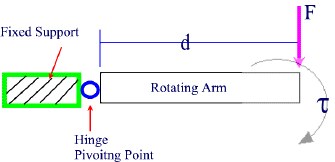#### Torque

Levers can be understood by applying the principle of Torque.

An object that rotates about a fixed point or about an axis because of a force being applied to it has Torque.
When a force is applied to a lever or rotating arm (an object attached to a fixed support by a pivoting point or hinge), the lever will move in a rotational motion.

The (angular) acceleration on the arm increases if

• a) the force  increases
• b) the length of the arm increases
The diagram below illustrates how a hinge works.
A small metal lever such as a hinge allows a bigger object such as a door to be easily opened or closed with little effort.The unit of torque is the Newton-meter [Nm].

Example:
If a 40 Kg rock needs to be raised using a 3.0 m lever, what will the resulting torque be on the rock?

Given: m = 40 Kg   d = 3.0 m    g = 9.8 N/Kg

Find:  Torque

need to find Fg first

Fg = m x g

= 40 Kg x 9.8 N/Kg

= 392 N

Solution:

Torque   t

t = F x d

= 392 N x 3.0 m

= 1176 Nm